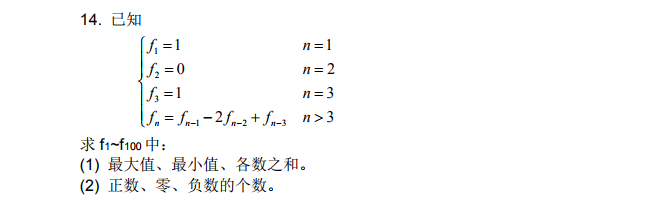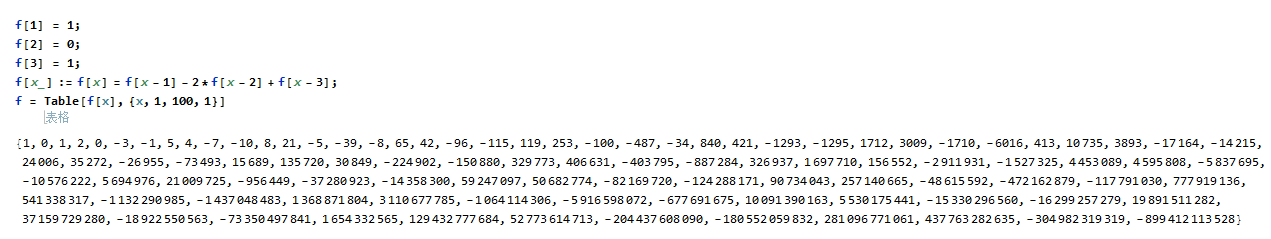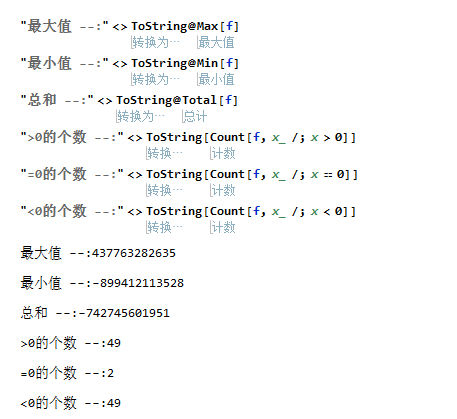# Mathematica入门习题–条件(;/)的使用

• A+

## 第十四题``````(*求出f1-f100的值*)
f = 1;
f = 0;
f = 1;
f[x_] := f[x] = f[x - 1] - 2*f[x - 2] + f[x - 3];
f = Table[f[x], {x, 1, 100, 1}]````````````"最大值 --:" <> ToString@Max[f]
"最小值 --:" <> ToString@Min[f]
"总和 --:" <> ToString@Total[f]
">0的个数 --:" <> ToString[Count[f, x_ /; x > 0]]
"=0的个数 --:" <> ToString[Count[f, x_ /; x == 0]]
"<0的个数 --:" <> ToString[Count[f, x_ /; x < 0]]``````• 微信公众号
• 关注微信公众号
•• QQ群
• 我们的QQ群号
•